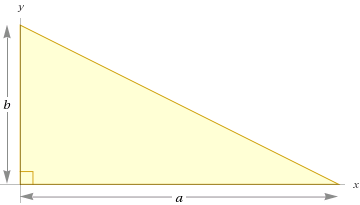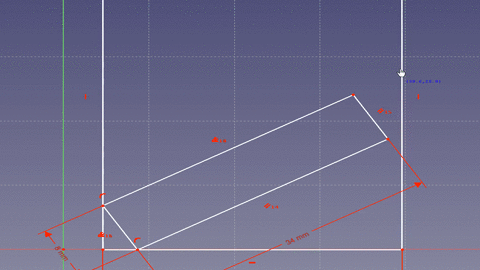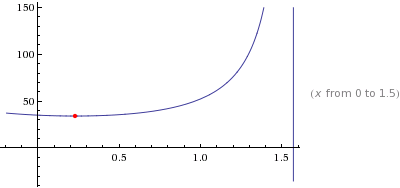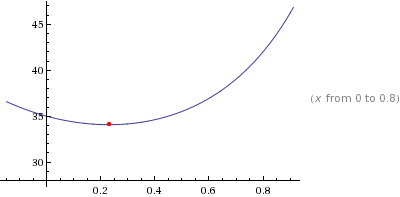Working on a personal project, I came across the need to cut a board such that I could rotate it into place inside a window sill. One could cut the board an inch or so sorter, try it out, and adjust as necessary, but what fun is that. Instead we can use math to find the answer!

After drawing out the problem, labeling the knowns and unknowns, I started playing around with the problem for a bit trying to see what relationships I could draw. One can represent the problem as a simple right triangle with the bottom of the board as the hypotenuse, the bottom of the window, and a side of the window as the other sides of the triangle.Given an angle x you could determine the longest board that would fit (ie the hypotenuse). Given a function that represents the length of a board that would fit at a given angle, the minimum of the function could be found to determine the maximum length board that could make it all the way though. Cosine of the angle between the board and the bottom of the sill (x in the diagram) provides that relationship if the board were simply a line and can be written as follows.

``````cos(x) = window width (a) / board length (c)
``````

Next one needs to account for the fact that the board is not just a line, but has width and when rotated the starting point of the board bottom is moved away from the window sill wall.That means the effective length of the window bottom (side a) in our equation is offset by some amount. Looking at the animation you can see that the corner of the board forms two right triangles. Since the bottom of the window is considered a continuous line there are 180 degrees (pi radians) on either side. The board takes up 90 degrees (pi/2 radians) leaving 90 degrees to split between the two angles made by the board with the bottom of the window. If we take the original equation and rewrite it to include the offset from the second triangle we get the following.

``````cos(x) = (window width - offset) / board length
``````

We can create an equation for the small triangle given that we know the hypotenuse is the width of the board we know the angle (z) is complementary to angle x and we are trying to solve for the adjacent side (offset).

``````cos(y) = offset / board width
``````

Solving for the offset and putting in terms of x:

``````y = (pi / 2) - x
cos((pi / 2) - x) = offset / board width
offset = board width * cos((pi / 2) - x)
``````

Plugging into the original equation with the offset and solving for board length.

``````cos(x) = (window width - (board width * cos((pi / 2) - x))) / board length
board length = (window width - (board width * cos((pi / 2) - x))) / cos(x)
``````

Graphed using the following (values for my window and board (1x8)).

``````window width = 35 inches
board width = 8 inches
minimize (35 - 8 * cos((pi / 2) - x))) / cos(x) over [0, pi/2]
``````A graph zoomed in to `[0, pi / 4]` better demonstrates the length change.The shortest length the board may be is not while the board is not while resetting flat against the window sill (x = 0 radians) which makes sense. Additionally, in the first graph you can see the board length goes out to infinity at pi/2 (90 degrees) which makes sense if you consider a board standing perfectly upright would never touch the other side of the window. The answer that Wolfram Alpha provides is:

``````board length = 34.0735 inches
``````

That means one should cut the board to 34 inches to be able to rotate it into place! For your convenience I have provided a widget which you can use to solve for your own use. Keep in mind this works for more than just windows, any rectangle object you wish to rotate into another rectangle.

For your convenience I created a widget to calculate board width given a window width.